September 9, 2015

# 使用虚幻引擎4可视化记录器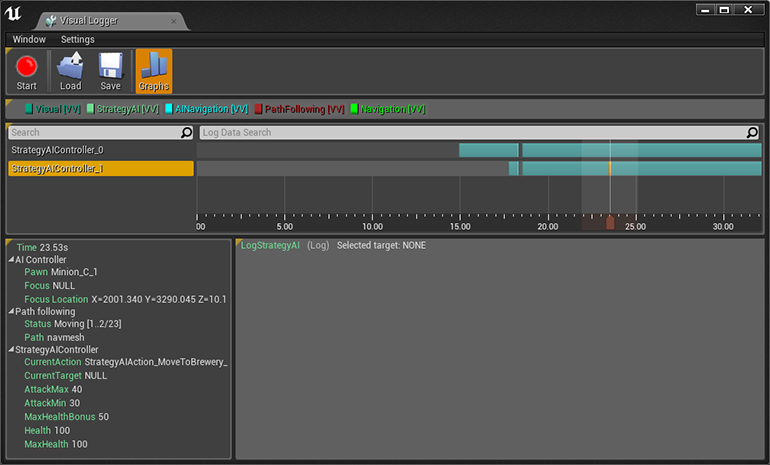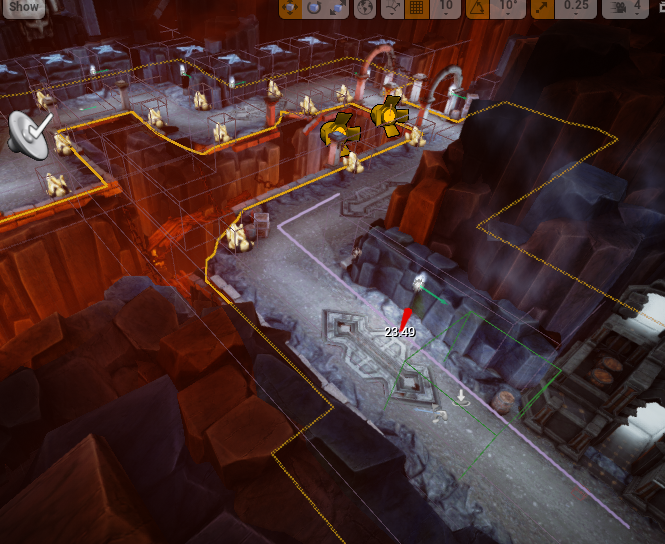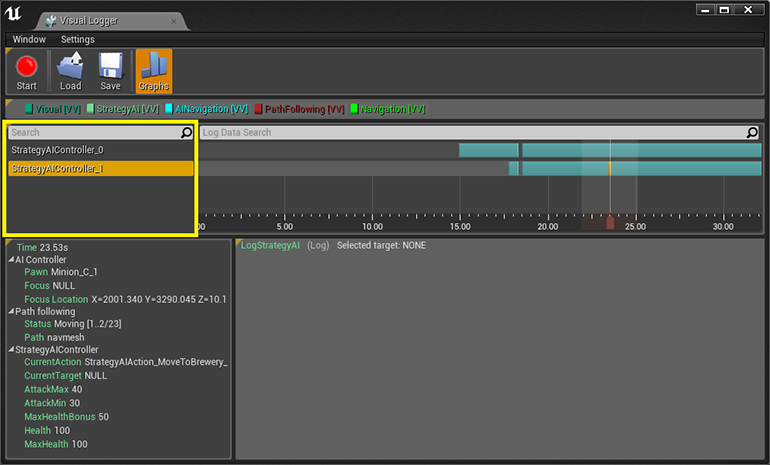Actor列表和搜索选项。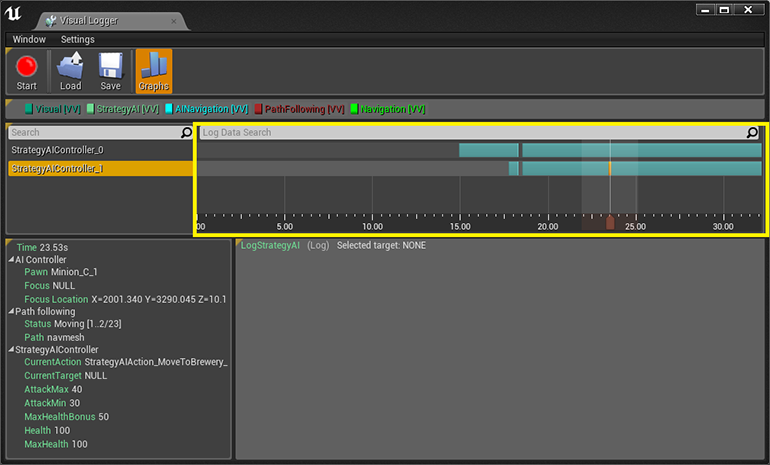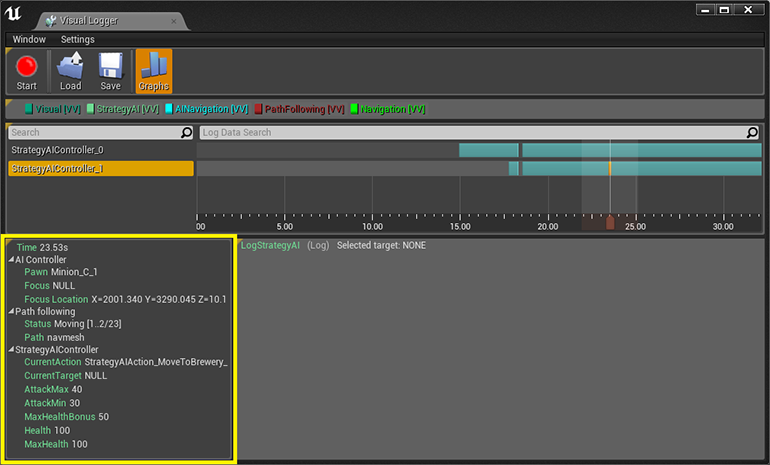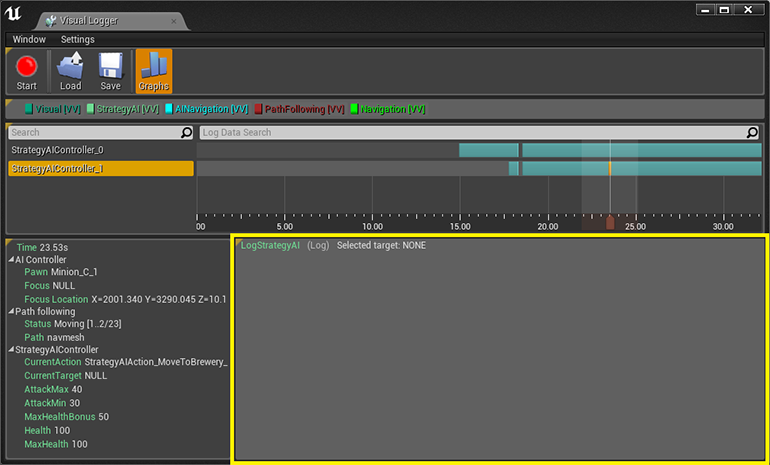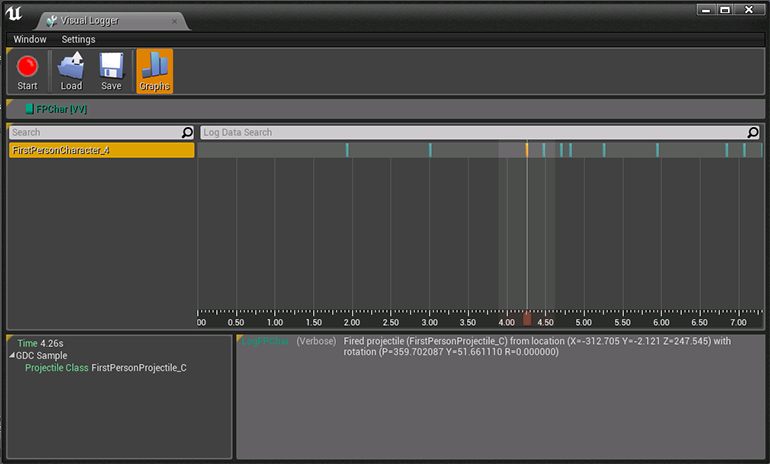`#if ENABLE_VISUAL_LOG`

`    /** Appends information about this actor to the visual logger */`

`    virtual void GrabDebugSnapshot(FVisualLogEntry* Snapshot) const override;`

`#endif`

`#if ENABLE_VISUAL_LOG`

`void AGDCCharacter::GrabDebugSnapshot(FVisualLogEntry* Snapshot) const`

`{`

`    Super::GrabDebugSnapshot(Snapshot);`

`    const int32 CatIndex = Snapshot->Status.AddZeroed();`

`    FVisualLogStatusCategory& PlaceableCategory = Snapshot->Status[CatIndex];`

`    PlaceableCategory.Category = TEXT("GDC Sample");`

`    PlaceableCategory.Add(TEXT("Projectile Class"), ProjectileClass != nullptr ? ProjectileClass->GetName() : TEXT("None"));`

`}`

`#endif`

`void AGDCCharacter::OnFire()`

`{`

`    // try and fire a projectile`

`    if (ProjectileClass != NULL)`

`    {`

`        const FRotator SpawnRotation = GetControlRotation();`

`        // MuzzleOffset is in camera space, so transform it to world space before offsetting from the character location to find the final muzzle position`

`        const FVector SpawnLocation = GetActorLocation() + SpawnRotation.RotateVector(GunOffset);`

`        UWorld* const World = GetWorld();`

`        if (World != NULL)`

`        {`

`            // spawn the projectile at the muzzle`

`            World->SpawnActor<AGDCProjectile>(ProjectileClass, SpawnLocation, SpawnRotation);`

`            UE_VLOG(this, LogFPChar, Verbose, TEXT("Fired projectile (%s) from location (%s) with rotation (%s)"),`

`                *ProjectileClass->GetName(),`

`                *SpawnLocation.ToString(),`

`                *SpawnRotation.ToString());`

`        }`

`    }`

`    // try and play the sound if specified`

`    if (FireSound != NULL)`

`    {`

`        UGameplayStatics::PlaySoundAtLocation(this, FireSound, GetActorLocation());`

`    }`

`    // try and play a firing animation if specified`

`    if(FireAnimation != NULL)`

`    {`

`        // Get the animation object for the arms mesh`

`        UAnimInstance* AnimInstance = Mesh1P->GetAnimInstance();`

`        if(AnimInstance != NULL)`

`        {`

`            AnimInstance->Montage_Play(FireAnimation, 1.f);`

`        }`

`    }`

`}`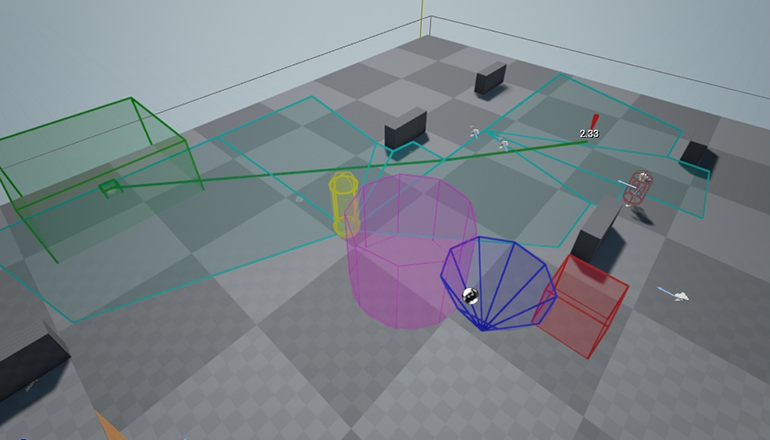• UE_VLOG_SEGMENT
• UE_VLOG_LOCATION
• UE_VLOG_BOX (axis aligned box)
• UE_VLOG_OBOX (oriented box)
• UE_VLOG_CONE
• UE_VLOG_CYLINDER
• UE_VLOG_CAPSULE